# (Solved):           Please assist with the following practice Questions ...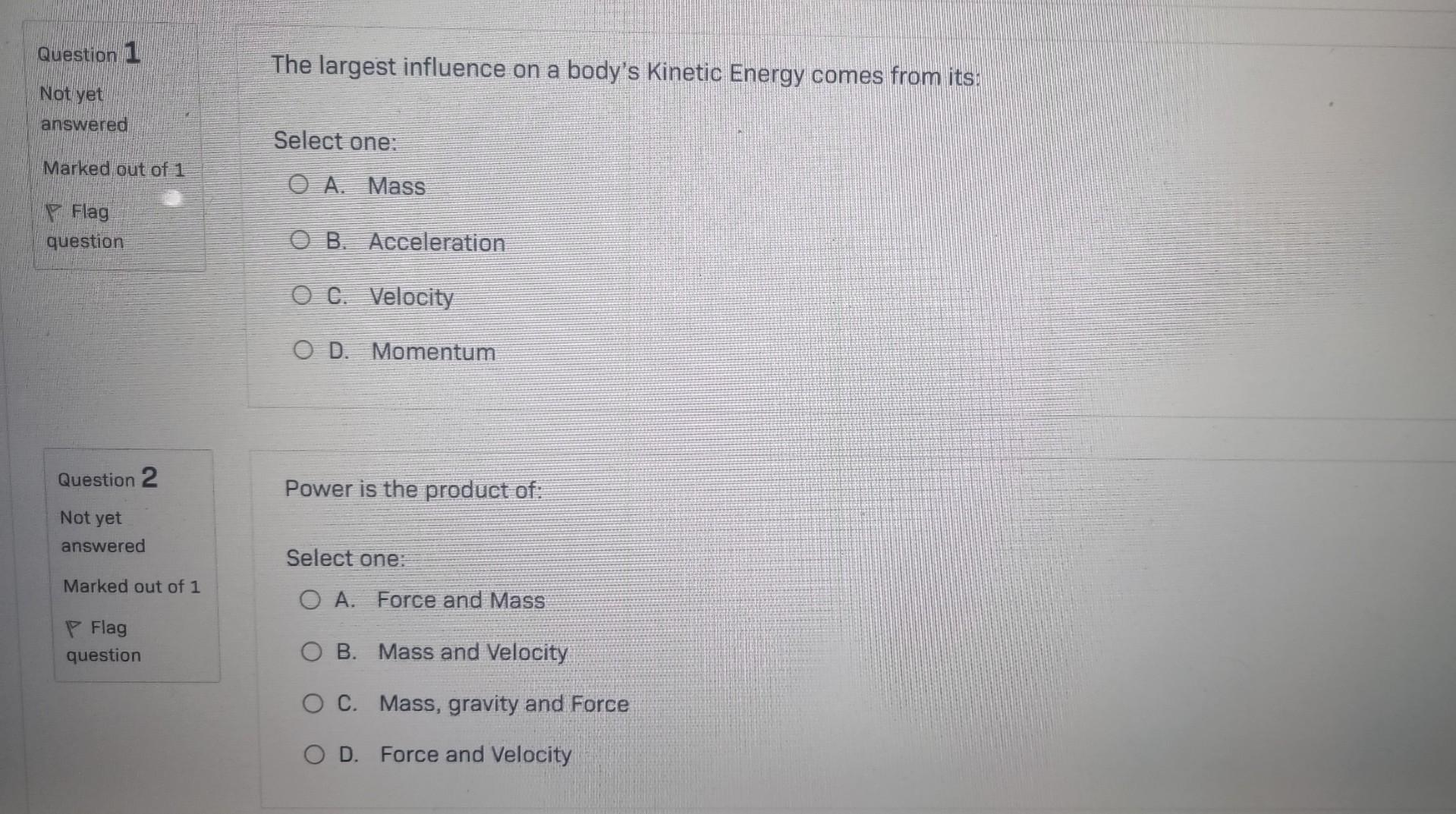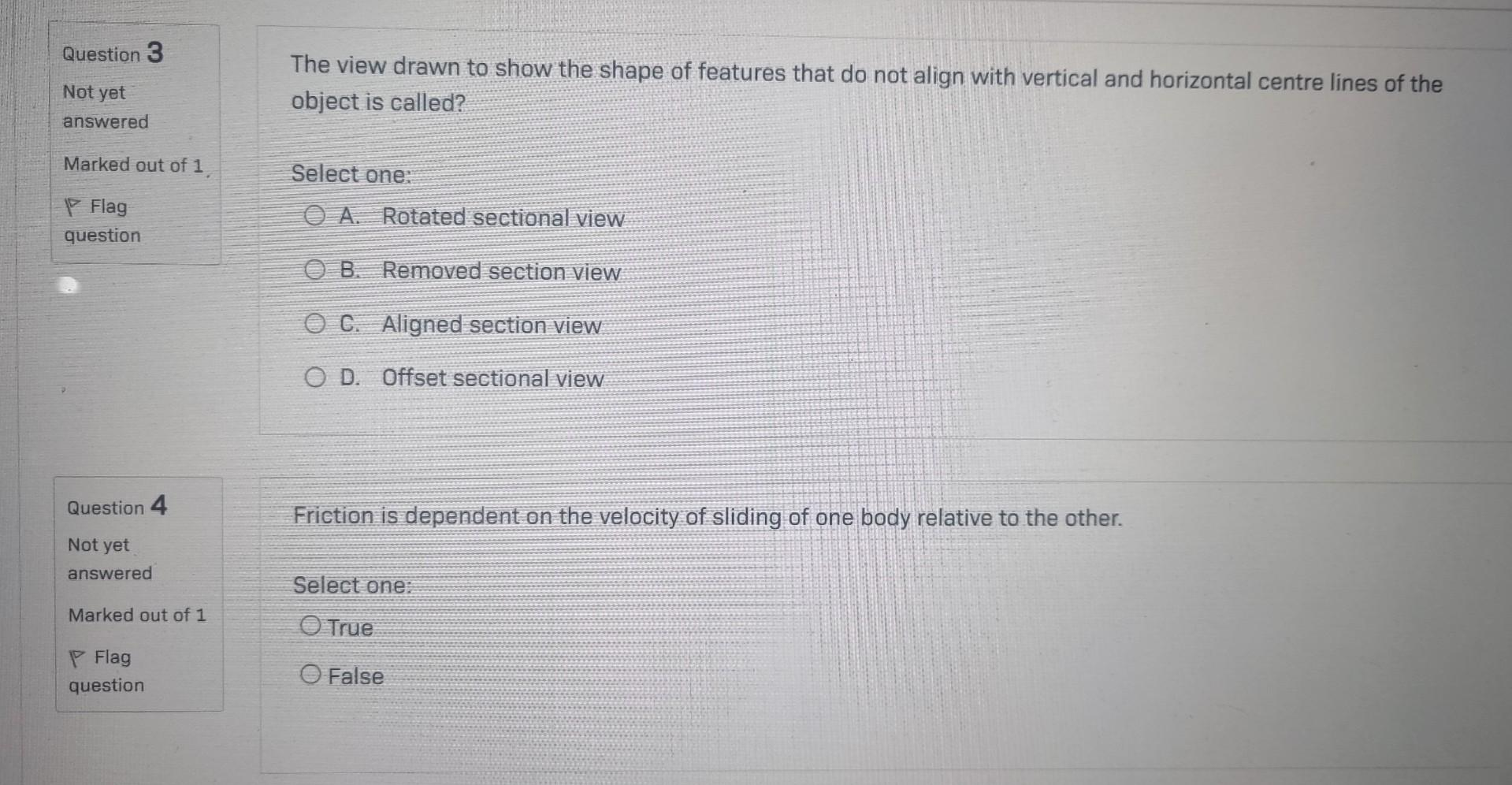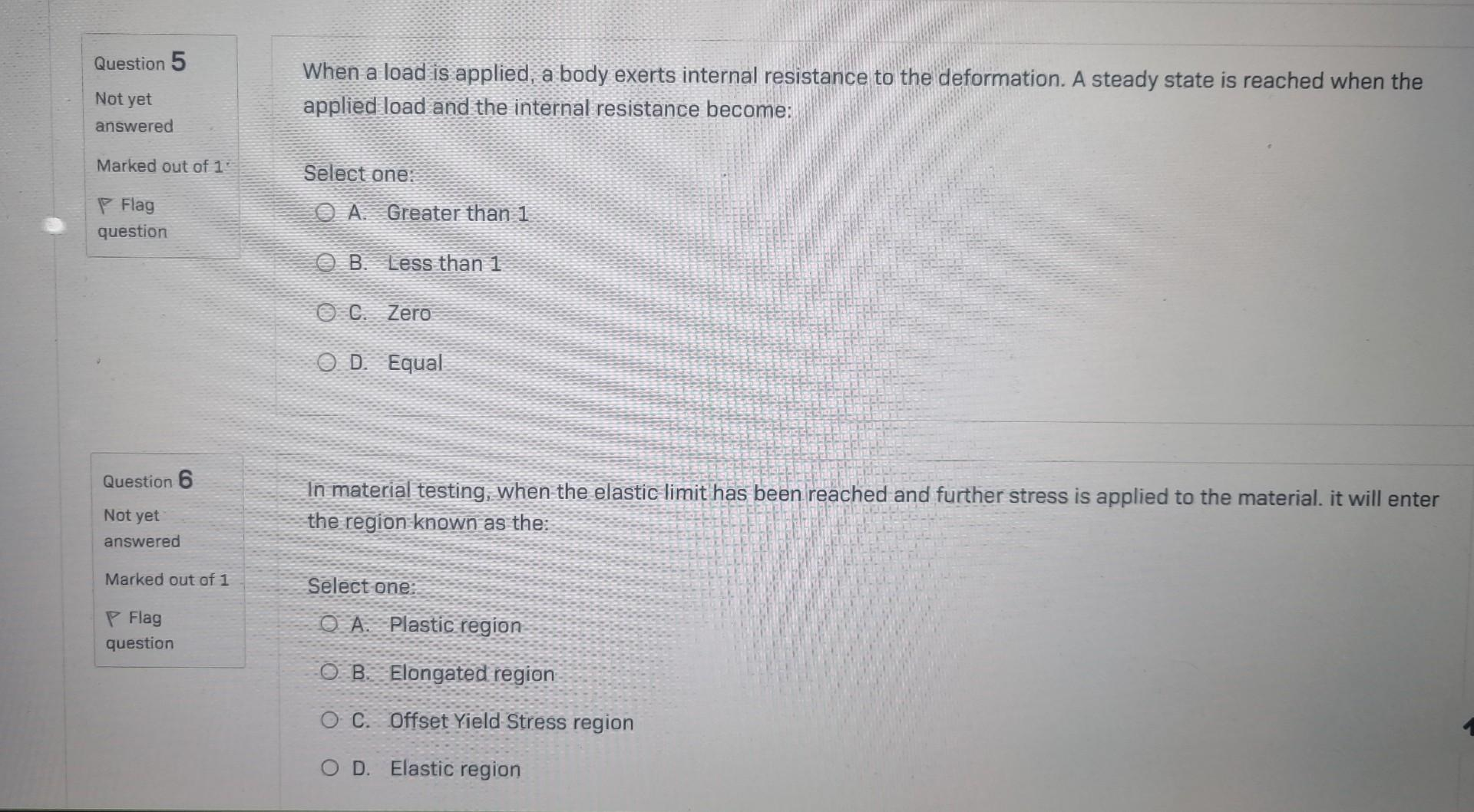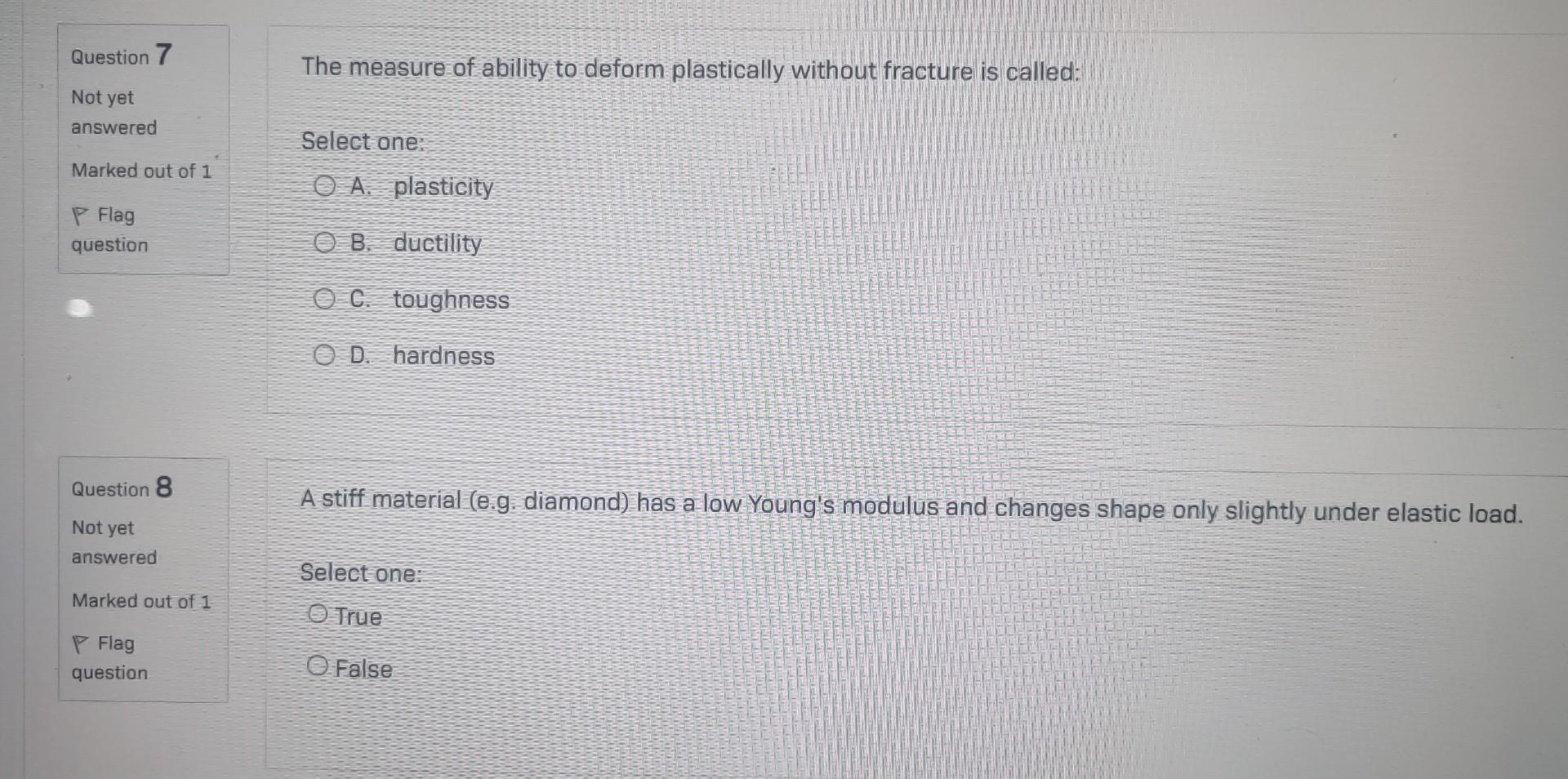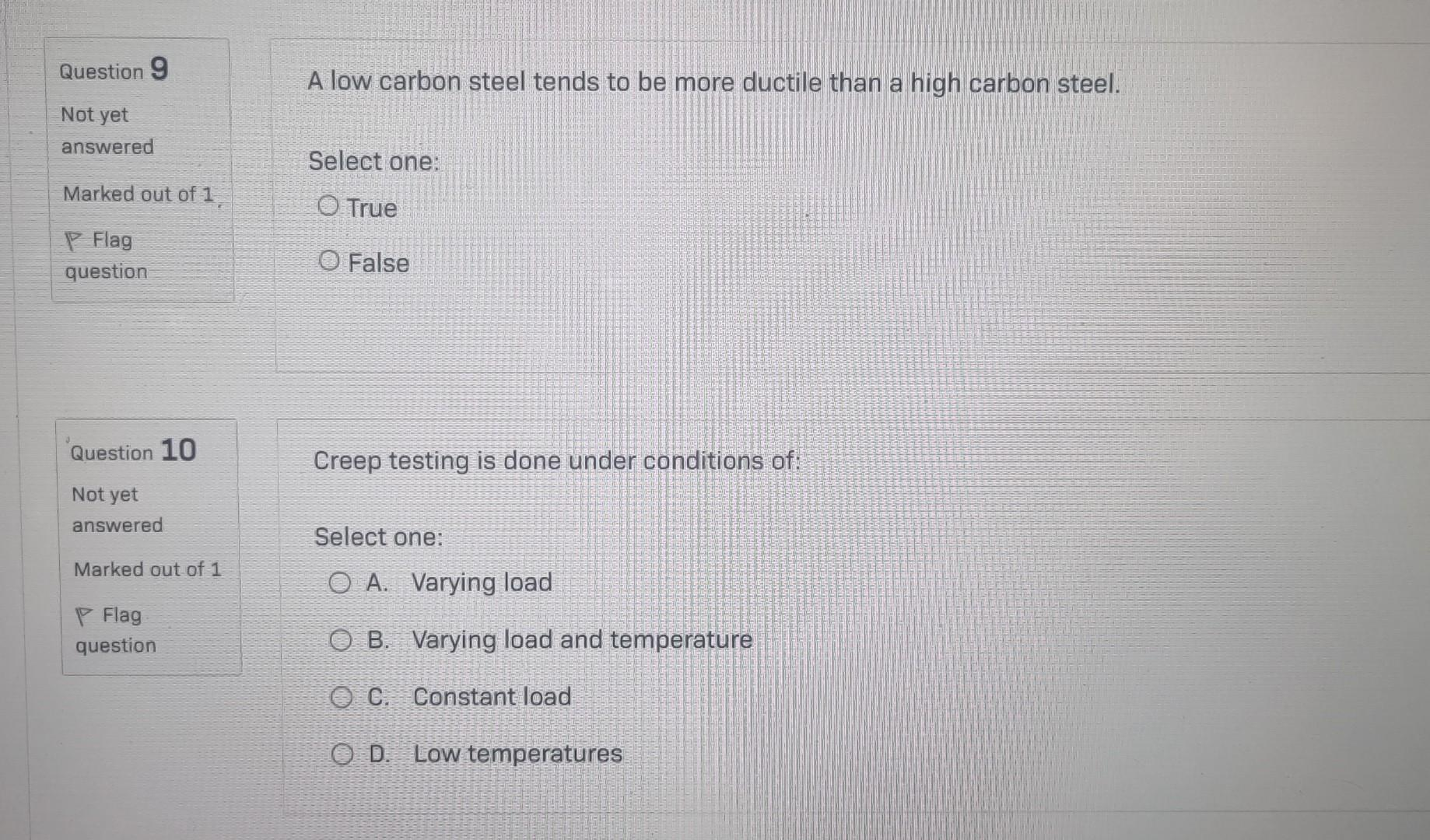Please assist with the following practice Questions ????

Not yet answered Select one: Marked out of 1 A. Mass P Flag B. Acceleration C. Velocity D. Momentum \begin{tabular}{|l} Question 2 \\ Not yet \\ answered \\ Marked out of 1 \\ P Flag \\ question \end{tabular} Power is the product of: Select one: A. Force and Mass B. Mass and Velocity C. Mass, gravity and Force D. Force and Velocity \begin{array}{ll}\text { Question } 3 & \text { The view drawn to show the shape of features that do not align with vertical and horizontal centre lines of the } \\ \text { Not yet }\end{array} Not yet answered Marked out of 1 . Select one: A. Rotated sectional view B. Removed section view C. Aligned section view D. Offset sectional view Question $$5 \quad$$ When a load is applied, a body exerts internal resistance to the deformation. A steady state is reached when the Not yet answered Marked out of 1 : Selectone: A. Greater than 1 B. Less than 1 C. Zero D. Equal In material testing, when the elastic limit has been reached and further stress is applied to the material. it will enter the region known as the Select one: A. Plastic region B. Elongated region C. Offset Yield Stress region D. Elastic region Not yet answered Select one: Marked out of 1 A. plasticity P Flag B. ductility C. toughness D. hardness A stiff material (e.g diamond) has a low Young's modulus and changes shape only slightly under elastic load. Selectone: Irue False Creep testing is done under conditions of: Select one: A. Varying load B. Varying load and temperature C. Constant load D. Low temperatures

We have an Answer from Expert